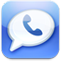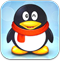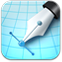# NOHKEN静电容量式物位开关•Features

Easy programming by push buttons

Multifunctional with microcomputer

First level switch to have status indicating LCD

Improved buildup prevention performance

Extremely reliable

Principle of OperationSet range is divided into 8 segments, and ON/OFF points are set for the segments. Hysteresis is also programmable.

The basic oscillator circuit is of the parallel resonance circuit with L (coil) and C (capacitance between the electrodes).

The oscillation frequency (f) of this circuit is : f = 1/2 π √LC.

The frequency without material around the sensor (f1) is: f1 = 1/2 π √LC, where C is the capacitance without material around the sensor (zero point).

With material around the sensor, the capacitance increases (C+ΔC), and the frequency (f2) is: f2 = 1/2 π √L(C+ΔC), where C+ΔC is the capacitance with material around the sensor (span point).

The sensor detects the frequency change from f1 to f2, and actuates the relay. The frequency range is divided into 8 segments (between zero and span), and the set and reset points assigned on a desired segment, which means high limit, low limit and hysteresis can be programmed.电话直呼在线客服在线留言